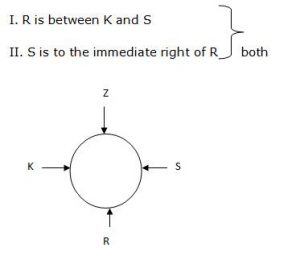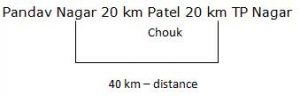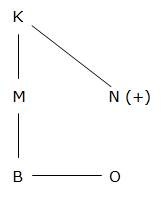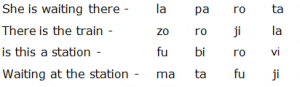## Coding and Decoding For SBI PO : Set – 39

D.1-5) Each of the questions below consists of a question and two statements I and II given below it.

1)  K,Z,R and S are sitting around a circle facing at the centre .Who is to the immediate Right of Z?

I. R is between K and S.

II. S is to the immediate right of R.

a) If the data in Statement I alone are sufficient to answer the question, while the data in statement II alone are not sufficient to answer the question

b) If the data in Statement II alone are sufficient to answer the question, while the data in statement I alone are not sufficient to answer the question

c) If the data either in statement I alone or in statement II alone are sufficient to answer the question

d) If the data given in both statements I and II together are not sufficient to answer the question

e) If the data in both statements I and II together are necessary to answer the question

e)If the data in both statements I and II together are necessary to answer the question

2) Mohan is older than Kamal and Sharad is younger than Aravind.Who among them is the Youngest?

I. Sharad is Younger than Mohan

II. Aravind is Younger than Kamal

a) If the data in Statement I alone are sufficient to answer the question, while the data in statement II alone are not sufficient to answer the question

b) If the data in Statement II alone are sufficient to answer the question, while the data in statement I alone are not sufficient to answer the question

c) If the data either in statement I alone or in statement II alone are sufficient to answer the question

d) If the data given in both statements I and II together are not sufficient to answer the question

e) If the data in both statements I and II together are necessary to answer the question

b)

M > K > A > S

Aravind is younger than Kamal

If the data in statements II alone are sufficient to answer the question, while the data is not sufficient to answer the question

3) What is the shortest distance between Pandavnagar and TP nagar?

I. Pandavnagar is 20 Km away from Patel Chowk

II. TP nagar is 20 Km away from Patel Chowk

a) If the data in Statement I alone are sufficient to answer the question, while the data in statement II alone are not sufficient to answer the question

b) If the data in Statement II alone are sufficient to answer the question, while the data in statement I alone are not sufficient to answer the question

c) If the data either in statement I alone or in statement II alone are sufficient to answer the question

d) If the data given in both statements I and II together are not sufficient to answer the question

e) If the data in both statements I and II together are necessary to answer the question

d)If the data given in both statements I and II together are not sufficient to answer the question . because the direction of those places are not given.

4) How is M related to N?

I.B is the daughter of M and sister of O.

II.N is the son of K who is B’s grandfather.

a) If the data in Statement I alone are sufficient to answer the question, while the data in statement II alone are not sufficient to answer the question

b) If the data in Statement II alone are sufficient to answer the question, while the data in statement I alone are not sufficient to answer the question

c) If the data either in statement I alone or in statement II alone are sufficient to answer the question

d) If the data given in both statements I and II together are not sufficient to answer the question

e) If the data in both statements I and II together are necessary to answer the question

d)

How is M related to NIf the data given in both statements I and II together are not sufficient to answer the question

5) What is the code for ‘not’ in the code language?

I. In the code language ‘do not go’ is written as la ra de

II. In the code language ‘go to school’ is written as ka ma ra

a) If the data in Statement I alone are sufficient to answer the question, while the data in statement II alone are not sufficient to answer the question

b) If the data in Statement II alone are sufficient to answer the question, while the data in statement I alone are not sufficient to answer the question

c) If the data either in statement I alone or in statement II alone are sufficient to answer the question

d) If the data given in both statements I and II together are not sufficient to answer the question

e) If the data in both statements I and II together are necessary to answer the question

d)

From both the statements we could not find the code for ‘not’

D.6-10) Study the following information carefully to answer the given questions.

In a certain code, ‘she is waiting there’ is written as ‘la pa ro ta’, ‘there is the train’ is written as ‘zo ro ji la’, ‘waiting at the station’ is written as ‘ma ta fu ji’ and ‘is this a station’ is written as ‘fu bi ro vi’.

6) Which of the following may represent guard is waiting?

a) ro ta zo

b) ta ki ro

c) fu zo ki

d) ta ro ji

e) la ma ro

b)is – ro ,waiting – ta  , only ki is a new code

7) What is the code for at?

a) ma

b) ji

c) fu

d) ta

e) Cannot be determined

a)(a) at – ma

8) Which of the following represents the train station?

a) zo la ma

b) fu ji ta

c) fu ji zo

d) ro zo fu

e) Cannot be determined

c)the – ji , train – zo , Station – fu

9) What does la stand for?

a) is

b) train

c) waiting

d) the

e) there

e)10) What is the code for she?

a) la

b) pa

c) ro

d) ta

e) Either la or zo